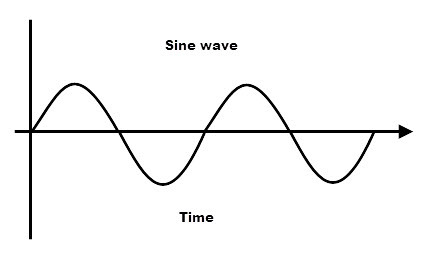# sinh() function in C++ STL

The sinh() is an inbuilt function in C++ STL which returns the hyperbolic sine of an angle given in radians.Syntax :

```sinh(data_type x)
```

Parameter :The function accepts one mandatory parameter x which specifies the hyperbolic angle in radian. The parameter can be of double, float or long double datatype.

Return Value: The function returns the hyperbolic sine of the argument. If the magnitude of the result is too large to be represented by a value of the return type, the function returns inf.

Program 1:

 `// C++ program to demonstrate the ` `// sinh() function ` `#include ` `using` `namespace` `std; ` ` `  `int` `main() ` `{ ` `    ``double` `x = 4.1; ` ` `  `    ``double` `result = ``sinh``(x); ` `    ``cout << ``"sinh(4.1) = "` `<< result << endl; ` ` `  `    ``// x in Degrees ` `    ``double` `xDegrees = 90; ` `    ``x = xDegrees * 3.14159 / 180; ` ` `  `    ``result = ``sinh``(x); ` `    ``cout << ``"sinh(90 degrees) = "` `<< result << endl; ` ` `  `    ``return` `0; ` `} `

Output:

```sinh(4.1) = 30.1619
sinh(90 degrees) = 2.3013
```

Program 2:

 `// C++ program to demonstrate the ` `// sinh() function ` `#include ` `using` `namespace` `std; ` ` `  `int` `main() ` `{ ` `    ``int` `x = -4; ` ` `  `    ``double` `result = ``sinh``(x); ` `    ``cout << ``"sinh(-4) = "` `<< result << endl; ` ` `  `    ``// x in Degrees ` `    ``double` `xDegrees = 90; ` `    ``// convert to radians ` `    ``x = xDegrees * 3.14159 / 180; ` ` `  `    ``result = ``sinh``(x); ` `    ``cout << ``"sinh(90 degrees) = "` `<< result << endl; ` ` `  `    ``return` `0; ` `} `

Output:

```sinh(-4) = -27.2899
sinh(90 degrees) = 1.1752
```

Errors and Exceptions: The function returns no matching function for call to error when a string or character is passed as an argument.

Below programs illustrate the errors and exceptions of sinh() method:

Program 3:

 `// C++ program to demonstrate the  ` `// sinh() function when a string is passed ` `#include ` `using` `namespace` `std; ` ` `  `int` `main() ` `{ ` `    ``string x = ``"gfg"``; ` `    ``double` `result; ` ` `  `    ``result = ``sinh``(x); ` `    ``cout << ``"sinh(x) = "` `<< result << endl; ` ` `  `    ``return` `0; ` `} `

Output:

```prog.cpp:14:20: error: no matching function for call to 'sinh(std::__cxx11::string&)'
result = sinh(x);
```

Program 4:

 `// C++ program to demonstrate the sinh() ` `// function When argument is too large ` `#include ` `using` `namespace` `std; ` ` `  `int` `main() ` `{ ` `    ``double` `x = 3000.0;  ` ` `  `    ``double` `result = ``sinh``(x); ` `    ``cout << ``"sinh(x) = "` `<< result << endl; ` ` `  `    ``return` `0; ` `} `

Output:

```sinh(3000.0) = inf
```

My Personal Notes arrow_drop_upCheck out this Author's contributed articles.

If you like GeeksforGeeks and would like to contribute, you can also write an article using contribute.geeksforgeeks.org or mail your article to contribute@geeksforgeeks.org. See your article appearing on the GeeksforGeeks main page and help other Geeks.

Please Improve this article if you find anything incorrect by clicking on the "Improve Article" button below.

Improved By : mathemagic

Article Tags :
Practice Tags :

Be the First to upvote.

Please write to us at contribute@geeksforgeeks.org to report any issue with the above content.